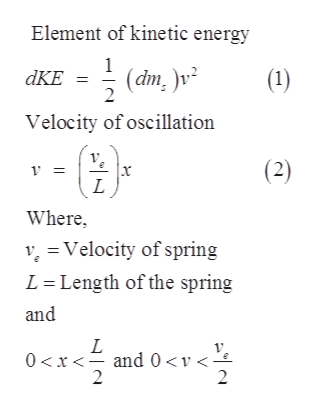Question
15 views

We will now determine how the 1/3 rule comes about.

Consider a spring of mass ms which is attached to a wall and oscillates on a frictionless surface as shown below. The spring’s mass is uniformly distributed along the length of the spring.

We will start with the infinitesimal form of kinetic energy, i.e. dKE = ½ (dms )v2. This formula will apply to an infinitesimal segment of the spring of length dx and mass dms as indicated below.

For any point on the spring, the velocity of oscillation will be given by v = (ve/L)x where ve is the velocity of the spring at its end where the mass m is attached, and L is the stretched length of the spring at that instant. Thus, when x = 0 then v = 0, and when x = L/2 then v = ½ ve.

Hint: Figure out how to relate dms to dx and then integrate both sides of the infinitesimal kinetic energy equation to get an equation for the kinetic energy of the spring that includes ms/3.

check_circle

Step 1

Given,help_outlineImage TranscriptioncloseElement of kinetic energy 1 (dm, )v2 dKE Velocity of oscillation (2) L Where vVelocity of spring L Length of the spring and L 0 x and 0
Step 2

FBD:

Step 3

Let the dm is mass element and dx is the...

### Want to see the full answer?

See Solution

#### Want to see this answer and more?

Solutions are written by subject experts who are available 24/7. Questions are typically answered within 1 hour.*

See Solution
*Response times may vary by subject and question.
Tagged in

### Physics